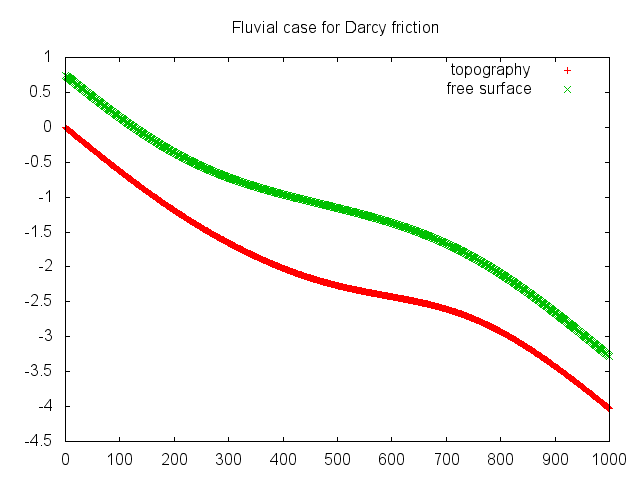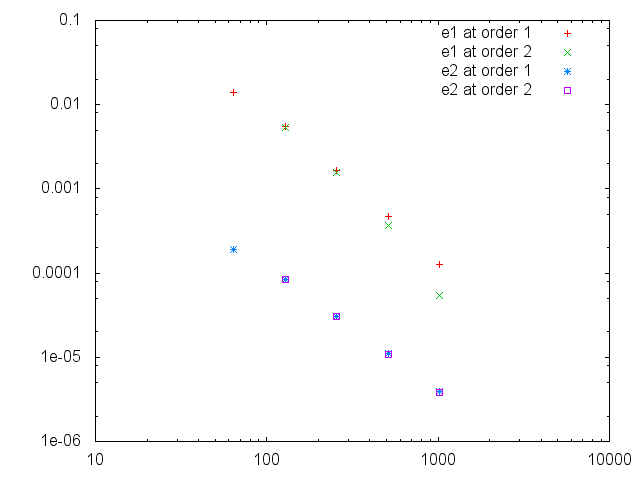# Benchmarks

## Friction test cases

Here we test the friction source term in the case of fluvial/torrential flows for three differents source terms. The original test case is the one of MacDonald and you can find more similar test cases in Delestre & al.. The principle is to set the topography in order to have an analytical solution for h(x). The source term is treated semi-implicitly at the same order than the Saint-Venant function, which is at order 2, except for the Darcy 1 order case.

### Case description

We consider a 1D topography z_b(x) which is 1000 metres long. At steady state, the momentum equation of Saint-Venant can be written as :

\displaystyle \partial_x z_b(x) = (\frac{q^2}{g h^3}-1) \partial_x h(x) - S_f \ ,

where S_f is the friction term. By chosing an analytical function hex(x) for h(x), we can find the resulting topography with a r-k method.

### Fluvial

For the sub-critical case, the analytical water height at steady state is :

\displaystyle hex(x) = \left( \frac{4}{g} \right)^{1/3} \left( 1 + 0.5\times exp\left( -16\times (\frac{x}{1000}-0.5)^2 \right) \right).

The slope is initially dry (h(x) = u(x) = 0) and we chose as boundaries conditions :

\displaystyle q[left] = 1.5 m^2 .s^{-1} \ and \ h[left] = hex(0) ,

\displaystyle q[right] = 1.5 m^2 .s^{-1} \ and \ h[right] = hex(1000).

We plot an example of the resulting topography for the Darcy friction :### Torrential

For the super-critical case, the analytical water height at steady state is :

\displaystyle hex(x) = \left( \frac{4}{g} \right)^{1/3} \left( 1 - 0.2\times exp\left( -36\times (\frac{x}{1000}-0.5)^2 \right) \right).

The slope is initially dry (h(x) = u(x) = 0) and we chose as boundaries conditions :

\displaystyle q[left] = 2.5 m^2 .s^{-1} \ and \ h[left] = hex(0).

We plot an example of the resulting topography for the Darcy friction :### Order treatment

We compare the convergence of |h|_1 for the different source treatment (order 1 and 2), for the torrential case with the darcy friction term :Error Convergence

Note that the convergence depends strongly on the chosen source term.

### Subcritical to super-critical

The flow is subcritical upstream and super-critical downstream. The water height is :

\displaystyle h_{ex}(x) = \left( \frac{4}{g} \right)^{1/3} \left( 1 - 3\times tanh\left( -3\times (\frac{x}{1000}-0.5)^2 \right) \right). for 0 < x < 500 and : \displaystyle h_{ex}(x) = \left( \frac{4}{g} \right)^{1/6} \left( 1 - 6\times tanh\left( -3\times (\frac{x}{1000}-0.5)^2 \right) \right). for 500 < x < 1000.

• Darcy with q0 = 2\ m. s^{-1} and f = 0.042

## Rain test cases

We add a rain term to the MacDonald test case, as seen in Delestre et al.. Write R the constant rain intensity, the flux along the channel is written :

\displaystyle q(x) = q0 + x*R.

So the topography is now :

\displaystyle \partial_x z_b(x) = (\frac{q(x)^2}{g h^3}-1) \partial_x h(x) -\frac{2q(x) R}{g h(x)^2}- S_f

We take the same function for hex(x) seen with the friction test cases in both torrential and fluvial case and we use the Darcy friction term :

 I. MacDonald, M. Baines, N. Nichols, and P. G. Samuels, “Analytic Benchmark Solutions for Open-Channel Flows,” no. November, pp. 1041–1045, 1997.

 O. Delestre, C. Lucas, P. A. Ksinant, F. Darboux, C. Laguerre, T. N. T. Vo, F. James, and S. Cordier, “SWASHES: A compilation of shallow water analytic solutions for hydraulic and environmental studies,” Int. J. Numer. Methods Fluids, vol. 72, pp. 269–300, 2013.